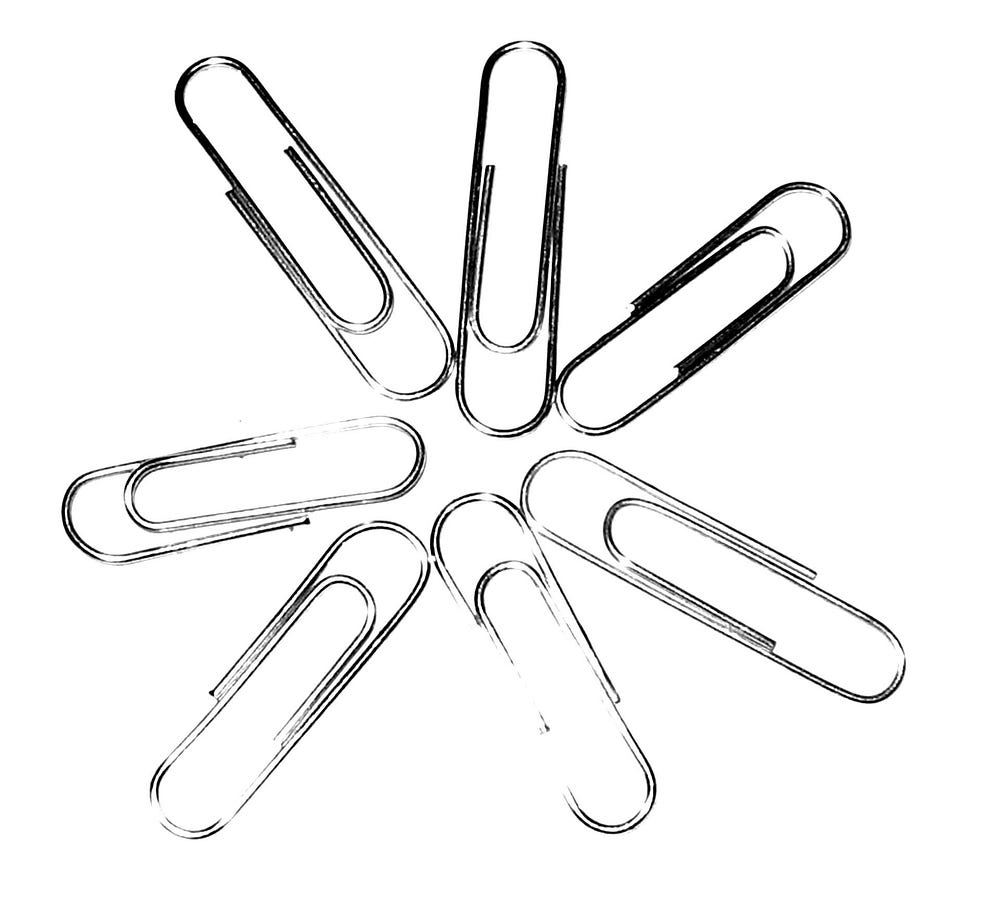# The paperclip maximizer won’t maximize paperclips

Original article can be found here (source): Artificial Intelligence on MediumWhy training is not training

We need to understand how we train machine learning models to understand how we would build a paperclip maximizer. A machine learning system is a mathematical model with lots of tweakable parameters, like dials and knobs on some giant machine. These parameters generally take the form of some number, and can be increased or decreased to change the output’s model. For this article we will focus on neural networks, since those yield some of the best machine learning results to date. To “train” a machine learning program, you tweak its parameters so that the model’s outputs get closer to what you want. There are three main ways to do this: backpropagation, reinforcement learning, and neuro-evolution.

1. Backpropagation

Backpropagation is the most common technique for training models and is useful when you have a defined set of expected output values for a set of input values. An example of when you would use backpropagation would be a model that predicts tomorrow’s weather off a set of inputs, such as the weather from the last few days. By tracking the weather for a while, we can create a dataset of inputs and outputs. We then pass the inputs to the model and see how different the model’s output is from what we expected the output to be in our dataset. This difference between the expected and actual output is called the error. After that, we adjust the model’s parameters to reduce the error. That’s basically it.

2. Reinforcement Learning

For reinforcement learning we allow the model to act in an environment, and then we adjust values depending on its behavior. Let’s consider a model that plays a video game. We allow the model to act semi-randomly at first, but whenever it wins the game, we update the parameters of the model so that it does more of whatever it did to win the game. This improves the performance of the model over time.

3. Neural Evolution

Lastly, for neural evolution, we create multiple models with different initial parameters. Naturally, some will perform better than others at the task. We then take the best ones and create new models by “mutating” our best models. This mutation is simply the random adjustment of some of the models’ parameters. We then repeat this, creating a selection process where only the best mutations are kept.

The key insight here is that we are not literally training anything. We are adjusting numbers in a mathematical model so that it minimizes an error function. It may be helpful to imagine this in terms of some human analogue, such as training or education, but this is just confusing the issue.

Now that we understand that “training” a model is just tweaking parameters, it is important to highlight that updating the model’s parameters will almost surely never create a perfect model.

When we adjust a model’s parameters to improve its performance, we adjust them in the direction that appears to lower the error. The problem is that we often get stuck in local minima. In the below picture, the X axis represents some parameter of our machine learning model, and the Y axis represents the error. Our goal is to adjust our parameter bit by bit in whichever direction reduces the error. Once we find ourselves in a local minimum however, it is very hard to get out, since no matter which way we go our error will increase.

If you are wondering why we don’t just set the parameter to the value which yields the global minimum, it is because we usually don’t know what that value is. If we knew the optimal value of every parameter, we would not need to go through this evolution/training process. Because of this, the behavior of a model will never perfectly line up with the selection process used to train it.